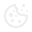# Improving performance with parallel loops

When carrying out computationally heavy calculations within a loop, dramatic performance improvements can be made by switching to a parallel loop. I recently implemented a parallel for each loop in order to benefit from the performance improvements. In this case study I outline what I learned, and some pitfalls to beware of.

During the programming of an e-commerce system I had to undertake some quite complicated calculations on a lot of products to calculate the price. Originally these calculations were in a foreach loop, but when I changed to a parallel foreach loop the time savings were dramatic. A parallel foreach loop takes longer to set up than a simple foreach loop, so it is slower when looping a small number of times. However when it has to loop a lot of times you can see really big performance improvements. The following table illustrates this by showing the time for the loop to complete using the same query but changing the number of products which the price was being calculated for:

Number of Products

1
50
230

Time for each loop (seconds)

87.86
0.45
18.28

Time parallel for each loop (seconds)

0.57
6.82
27.47

Getting Started
Switching from a foreach loop to a parallel foreach loop is really simple. For example given the following foreach loop:

``````foreach (var product in products)
{
CalculatePrice(product);
}``````

Write the same loop using a parallel foreach loop as so:

``````Parallel.ForEach(products, product =>
{
CalculatePrice(product);
)};``````

That is all it takes to convert a foreach loop to a parallel foreach loop.

Although it is very simple to write a parallel foreach loop, you cannot simply paste in the code from a normal foreach loop and expect it to simply work. Because the parallel loop is running in different threads all of the code inside the loop must be threadsafe. This means that the code functions the same way even if multiple threads are running at the same time.

Shared Variables
The first thing to be careful of with a parallel loop are shared variables, shared variables should never be used. For example the following code is dangerous:

``````decimal price;
Parallel.ForEach(products, product =>
{
string displayPrice = product.Name + " CHF";
price = CalculatePrice(product);
displayPrice = displayPrice + displayPrice;
)};``````

If there are 2 products: an apple with a price of CHF 2 and an apple macbook with a price of CHF 1000. Then what could happen is the display price string is for the apple and the price is calculated as 2. But then before the next line of code is executed the second thread sets the display price to 1000, so now you get the displayPrice string = apple CHF 1000 which is wrong. This code is not threadsafe.

Locks

``````List<decimal> prices;
object priceLock = new object();
Parallel.ForEach(products, product =>
{
decimal price = CalculatePrice(product);
lock(priceLock)
{
}
)};``````

Obviously using locks slows down the code, because the code must execute synchronously inside the lock, so they should only be used when needed.

Interlocked
Interlocked is especially for commands which are not normally thread safe, but are commonly used in parallel programming. One of these is the ++ operator. Normally this operator is not atomic and therefore not thread safe and would have to be put inside a lock. But Microsoft have implemented the same logic using Interlocked. Increment which is thread safe, and so can be used safely outside of a lock in a parallel loop. Below is an example using interlocked increment:

``````List<decimal> prices;
object priceLock = new object();
int totalPricesCalculated = 0;
Parallel.ForEach(products, product =>
{
decimal price = CalculatePrice(product);
lock(priceLock)
{
}
Interlocked.Increment(ref totalPricesCalculated);
)};``````

Data access
In our project we use entity framework to access the database. However the entity framework context is not thread safe. So I could not access the database within the parallel loop. Instead I made sure to fetch all the data needed for the price calculation before entering the loop from the database into memory, this then meant that the calculation could complete with no errors.

Debugging
It is not easy to debug a parallel loop. The problem is that with many different threads when stepping into the code the debugger seems to jump around as it moves from one thread to another both at different points in the code. The solution that I found most useful was to run the parallel loop synchronously for debugging, you can then step through the code and easily find any bugs without being confused. The parallel foreach loop makes this very easy by taking in options which you can set before the loop starts. This ParallelOptions object has a property MaxDegreeOfParallelism, If this is set to -1, then it uses as many threads as possible, but if it is set to 1, then the parallel foreach loop will behave synchronously, just the same as a normal foreach loop. Here is an example of using the parallel options to set the loop to run synchronously for easier debugging:

``````var options = new ParallelOptions { MaxDegreeOfParallelism = 1 };
Parallel.ForEach(products, options, product =>
{
decimal price = CalculatePrice(product);
)};``````

Conclusion
Using a parallel loop means you have to think more carefully about your code to make sure that it is thread safe, but the performance improvements make the effort worthwhile.

###Cookies

Wir verwenden Cookies zur Bereitstellung von Webseiten-Funktionen und zu Analysezwecken.Mehr Informationen